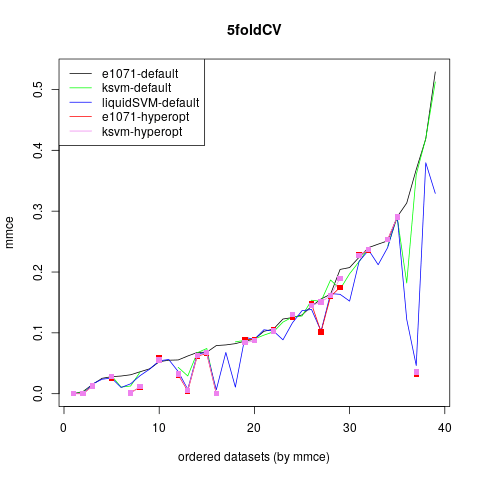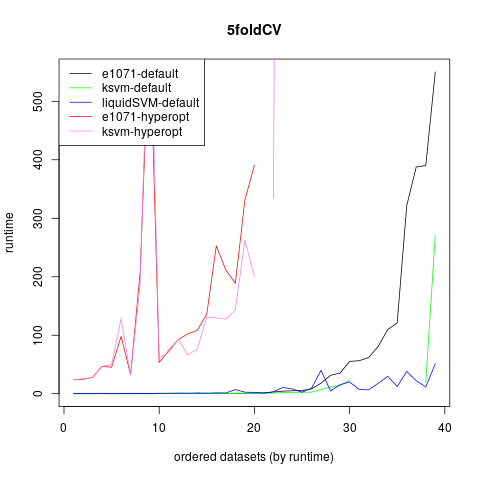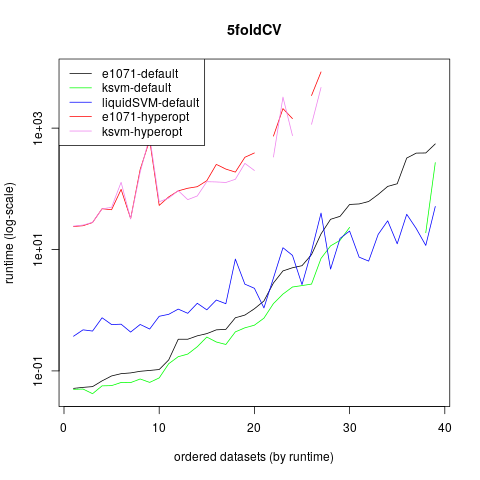Want to share your content on R-bloggers? click here if you have a blog, or here if you don't.

In this article I will present a benchmark analysis of different implementations of support vector machines in R. The three packages that I will compare are the most popular package e1071, the also well known package kernlab and the less well known package liquidSVM.

## Different packages

I will use 38 binary classification datasets of the OpenML100, that I already used for other benchmark analysis.

I compare these three packages in their default version. All packages use the radial kernel as default. In e1071 $\gamma$ is set to $1/p$ with $p$ being the number of observations and the cost parameter is set to 1. In kernlab the cost parameter is also set to 1 and $\gamma$ is set by an automatic procedure/heuristic that was developed by Caputo et. al. In liquidSVM as default a grid search on $\gamma$ and the cost parameter is executed with $\gamma \in [0.2,5]$ and the regularization parameter $\lambda \in [0.001,0.01]$.

Moreover I do tuning via model-based optimization (MBO) for e1071 and kernlab. For this I use the package mlrHyperopt that uses internally mlrMBO for tuning. I set the hyperparameter ranges according to the popular paper A practical guide to Support Vector Classifier, which means sampling $\gamma \in [2^{-15}, 2^3]$ and the cost parameter $C \in [2^{-5}, 2^{15}]$ (sampling with equal distribution from $[-15,3]$ and $[-5,15]$ respectively and transforming them via the function $2^x$).

For the 13 smallest datasets a 5-fold cross-validation with 10 repetitions is executed, for the others a 5-fold cross-validation. The mean missclassification error (also called error rate) can be seen in the following graph. Some results of the tuning variants are not shown, as they took too much time for this exploratory analysis.The default of e1071 is slightly worse than the default of kernlab. The two mlrHyperopt tuning variants are equal or better than these two defaults, in several datasets tuning provides a better error rate than the default version, which underlines the necessity of tuning the support vector machine. The liquidSVM implementation is nearly always equally good as the two tuning variants, which is not surprising as there is an internal tuning in the package.

The next graph shows the runtime of the different implementations.It can be seen, that the runtime of the tuning algorithms is ok for smaller datasets, but for bigger datasets it raises up extremely and makes the execution infeasible. The runtime of the default of kernlab is slightly better than for e1071. The liquidSVM is nearly as fast as the default of the other packages and increases less than the other defaults for bigger datasets. There are also parallelizing options in the package and some other options for big datasets, which makes this package extremely well suited for bigger datasets.

Here is also a graph showing the runtimes on a log scale:All in all I can warmly recommend the liquidSVM package. There are still some problems in the R-Version when using probability predictions, but hopefully they will be solved soon.

In the next article I will probably compare support vector machine with random forest and outline advantages and disadvantages of these two algorithms.